# Bishop-Phelps theorem

Consider a real Banach space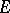, its (closed convex) unit ball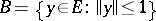, and its adjoint space of continuous linear functionals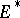(cf. Linear functional). If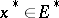, its norm is defined as its supremum on the closed convex set, that is,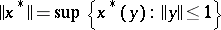. The fundamental Hahn–Banach theorem implies that if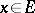and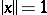, then there exists a continuous linear functionalsuch that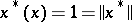. Thus, these "Hahn–Banach functionals" attain their suprema on, and by taking all positive scalar multiples of such functions, there are clearly "many" of them. The Bishop–Phelps theorem [a1] asserts that such norm-attaining functionals are actually norm dense in. (James' theorem [a4] shows that if every element ofattains its supremum on, thenis necessarily reflexive, cf. Reflexive space.) A more general Bishop–Phelps theorem yields the same norm density conclusion for the set of functionals inwhich attain their supremum on an arbitrary non-empty closed convex bounded subset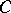of(the support functionals of). In fact, ifis any non-empty closed convex subset of, its support functionals are norm dense among those functionals which are bounded above on; moreover, the points ofat which support functionals attain their supremum on(the support points) are dense in the boundary of. (This contrasts with a geometric version of the Hahn–Banach theorem, which guarantees that every boundary point of a closed convex setis a support point, providedhas non-empty interior.)
This last result leads to the Brøndsted–Rockafellar theorem [a2], fundamental in convex analysis, about extended-real-valued lower semi-continuous convex functionsonwhich are proper, in the sense that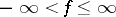and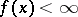for at least one point. The epigraphof such a function is a non-empty closed convex subset of the product space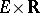(the real numbers) and the subgradients ofdefine support functionals of. The set of all subgradients toat(where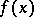is finite) form the subdifferential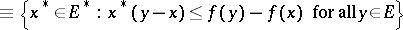ofat. The Brøndsted–Rockafellar theorem [a2] yields density, within the set of points whereis finite, of thosefor which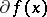is non-empty.